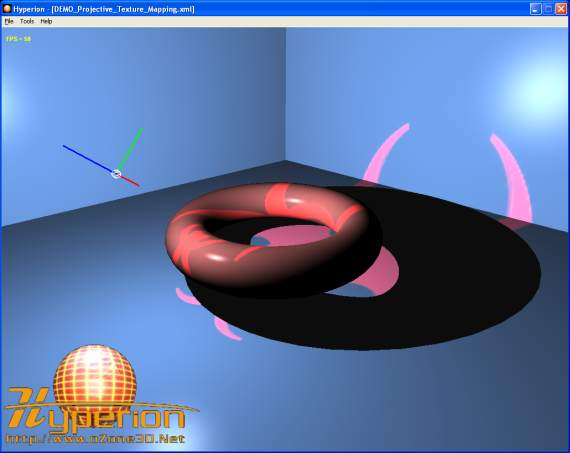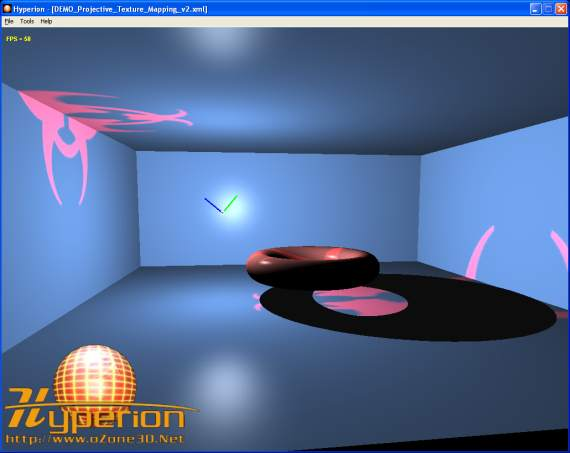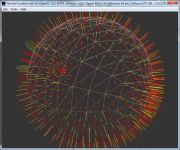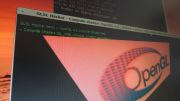Home Utilities Tutorials 3D Demos Graphics Cards Forums About

 GeeXLab
Current version: 0.29.17
>GeeXLab homepage

 FurMark
Current version: 1.21.1
>FurMark homepage

 GPU Caps Viewer
Current version: 1.44.2.1
>GPU Caps Viewer homepage

 GPU Shark
Current version: 0.16.1.0
>GPU Shark homepage

 Blogs
>JeGX's HackLab

 Geeks3D's Articles
>GPU Memory Speed Demystified

>How to Get your Multi-core CPU Busy at 100%

>How To Make a VGA Dummy Plug

>Night Vision Post Processing Filter

 PhysX FluidMark
Current version: 1.5.4
>FluidMark homepage

 TessMark
Current version: 0.3.0
>TessMark homepage

Current version: 0.3.0

 Demoniak3D
Current Version: 1.23.0
>Demoniak3D
>Libraries and Plugins
>Demos
>Codes Samples

3D Graphics Search Engine:The Art of Texturing Using The OpenGL Shading Language By Jerome Guinot aka 'JeGX' - jegx [at] ozone3d (dot) net Initial draft: April 15, 2006 [ Index ]»Next Page 8 - Projective Texture MappingThe projective texture mapping is a technique allowing a texture to be projected onto the scene's objects like a slide projector would do it. The secret of texture projection is based on the texture projection matrix initialization also called TexGenMatrix. If your 3D engine already performs projective texturing using the 3D pipeline fixed functions, the texGen matrix may be initialized by the following code: ```glActiveTextureARB( GL_TEXTURE0_ARB ); glTexGeni(GL_S, GL_EYE_PLANE, &TexGenMatrix); glTexGeni(GL_T, GL_EYE_PLANE, &TexGenMatrix); glTexGeni(GL_R, GL_EYE_PLANE, &TexGenMatrix); glTexGeni(GL_Q, GL_EYE_PLANE, &TexGenMatrix); ``` This matrix is accessible in the vertex shader by the gl_TextureMatrix[tu] variable where tu is the texture unit for which the TexGenMatrix matrix has been initialized (in the example, tu=0). For those that would like to implement the TexGenMatrix initialization, just understand that this matrix is somewhat equivalent to the camera matrix. Indeed, the texGen matrix defines all transformations (projection matrix, view matrix) of the projector that, in the texture projection context, works like a camera. For more detail on this topic, see the excellent whitepaper by Cass Everitt: Projective Texture Mapping. The DEMO_Projective_Texture_Mapping.xml demo shows all the stages of the texGen matrix construction for projective texture mapping. The construction of the texGen matrix being done using the great LUA language, the conversion to another language should be a mere formality! The following image shows the rendering of the DEMO_Projective_Texture_Mapping.xml demo:Fig. 22 - the DEMO_Projective_Texture_Mapping.xml demo The major advantage of making the texture projection with shaders is that the reverse projection can be solved in a simple and clean way. The following screenshot illustrates the reverse projection:Fig. 23 - the problem of reverse projection The projected texture coordinates are obtained by multiplying the TexGenMatrix by the vertex position in world space (cf posWorld in the code). These coordinates form a {s, t, r, q} quadruplet. The reverse projection occurs when the {q} coordinate becomes negative. It is not easy to act on the {q} coordinate at the fixed functions level, but in a pixel shader it is really simple as shown by the following shader code (from the DEMO_Projective_Texture_Mapping.xml demo): ```[Vertex_Shader] uniform mat4 TexGenMat; uniform mat4 InvViewMat; varying vec3 normal, lightDir, eyeVec; void main() { normal = gl_NormalMatrix * gl_Normal; vec4 posEye = gl_ModelViewMatrix * gl_Vertex; vec4 posWorld = InvViewMat * posEye; gl_TexCoord = TexGenMat * posWorld; lightDir = vec3(gl_LightSource.position.xyz - posEye.xyz); eyeVec = -posEye.xyz; gl_Position = ftransform(); } [Pixel_Shader] uniform sampler2D projMap; varying vec3 normal, lightDir, eyeVec; void main (void) { vec4 final_color = (gl_FrontLightModelProduct.sceneColor * gl_FrontMaterial.ambient) + (gl_LightSource.ambient * gl_FrontMaterial.ambient); vec3 N = normalize(normal); vec3 L = normalize(lightDir); float lambertTerm = dot(N,L); if(lambertTerm > 0.0) { final_color += gl_LightSource.diffuse * gl_FrontMaterial.diffuse * lambertTerm; vec3 E = normalize(eyeVec); vec3 R = reflect(-L, N); float specular = pow( max(dot(R, E), 0.0), gl_FrontMaterial.shininess ); final_color += gl_LightSource.specular * gl_FrontMaterial.specular * specular; // Suppress the reverse projection. if( gl_TexCoord.q>0.0 ) { vec4 ProjMapColor = texture2DProj(projMap, gl_TexCoord); final_color += ProjMapColor*lambertTerm; } } gl_FragColor = final_color; }``` This pixel shader is a variation of the phong lighting one available in the Point Light tutorial. The projected texture coordinates are calculated by the following code: ```vec4 posEye = gl_ModelViewMatrix * gl_Vertex; vec4 posWorld = InvViewMat * posEye; gl_TexCoord = TexGenMat * posWorld; ``` where TexGenMat is the texture projection matrix and InvViewMat is the inverse view matrix. The goal of this last one is simply to calculate the vertex position in world space. The texture projection is done in the pixel shader by the following code: ```if( gl_TexCoord.q>0.0 ) { vec4 ProjMapColor = texture2DProj(projMap, gl_TexCoord); final_color += ProjMapColor*lambertTerm; } ``` The texture2DProj() allows us to directly exploit the projected textures coordinates stored in gl_TexCoord. The call to texture2DProj() could be remplaced by a call to texture2D() but in that case we have to divide the {s, t} coordinates by the {q} one as the following code shows it: ```if( gl_TexCoord.q>0.0 ) { vec2 projCoords = gl_TexCoord.st / gl_TexCoord.q; vec4 ProjMapColor = texture2D(projMap, projCoords); final_color += ProjMapColor*lambertTerm; } ``` [ Index ]»Next Page

 GeeXLab demosGLSL - Mesh exploderPhysX 3 cloth demoNormal visualizer with GS